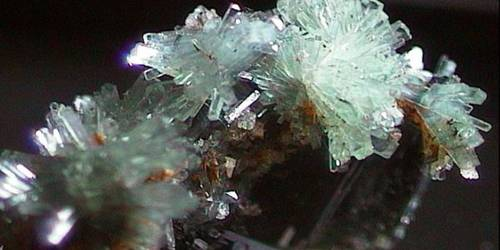Amicite has a general formula of K2Na2Al4Si4O16·5(H2O). It is a monoclinic-sphenoidal zeolite mineral containing aluminum, hydrogen, oxygen, potassium, silicon, and sodium. The name is in honor or Giovan Battista Amici (1786–1863) a physicist, optician, and inventor of the microscope optical elements.

General Information

• Category: Zeolite
• Formula: K2Na2Al4Si4O165(H2O)
• Crystal system: Monoclinic.

Structure and optical properties

Amicite is monoclinic, so the crystallography has three axes of unequal length and the angles between two of the axes are 90 degrees and one is less than 90. Amicite is also pseudotetragonal with a = 10.23, b = 10.43, c = 9.88, and d = 89, and belongs to the space group I2.Amicite is classified as biaxial anisotropic so the velocity of light varies depending on directly through the mineral, as well as showing double refraction. The index of refraction is the geometric ratio of the angle of light entering the crystal(angle of incidence) over the angle the light is bent when it enters the crystal (angle of refraction). The index of refraction can be defined mathematically as n=velocity of light in a vacuum/velocity of light in the mineral. Amicite has three indices of refraction na = 1.485, nb =1.490, nc = 1.494. A minerals birefringence is defined as the difference between the highest and lowest index of refraction, amicite’s birefringence is 0.009.

Properties

Amicite is a zeolite mineral, the commercial uses of zeolites are a function its three distinct properties: absorption, ion exchange, and catalysis. Zeolites are also known for their ability to absorb and lose water without any effect on its crystal structure.

• Formula mass: 690.51 g/mol
• Color: Colorless
• Twinning: none
• Cleavage: none
• Fracture: Conchoidal
• Luster: Vitreous
• Streak: White
• Diaphaneity: Transparent
• Specific gravity: 2.18
• Optical properties: Biaxial

Chemical composition

There are only two known occurrences of amicite, Hegau, Germany, and Khibiny massif, Russia. The framework composition of these two samples is TSi 0.51 and 0.49, respectively. In both samples, Na is approximately equal to K and Ca is very minor in amount. In both published analyses the H2O content is about 12.9 weight percent corresponding to 10 molecules per unit cell.

Information Source: